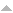Name: Carlos Eduardo Polatschek Kopperschmidt
Type: MSc dissertation
Publication date: 17/02/2020

Examining board:

NameRole
JOSÉ MIR JUSTINO DA COSTA External Examiner *
JULIO CESAR SAMPAIO DUTRA Co advisor *
Marcio Ferreira Martins Internal Examiner *
Wellington Betencurte da Silva Advisor *

Summary: Thermal processes related to most of practical problems involve the need to be investigated as inverse problems. In this aspect, the implementation of numerical or analytical-numerical solutions is essential because of complexity in obtaining purely analytical solutions, and this requires fast and accurate responses. This present work addresses, in the context of parabolic heat conduction problems, the Method of Fundamental Solutions (MFS) numerical approximations combined with Bayesian procedures for state and parameter estimating. In the MFS we consider the fundamental solution of the parabolic heat equation in order to solve the time-dependent term together with the resulting system of equations, without needing to perform que transformation of the Parabolic equation into Elliptic, therefore it does not require treating the time component separately. The cases presented consist of homogeneous problems whose solution is previously known, in order to assess the proposed method behavior for different situations. The investigated problems were based on Robin boundary to one and two-spatial dimensions and one dimension for the time. The method is easily extensible to higher dimension problems. Problems were also investigated whose contour is nonlinear, WHERE the nonlinearity is due to the presence of radiation in the system. The Bayesian method used in the inverse problems is based on the particle filter Sampling Importance Re-sampling (SIR), which is combined with the MFS to enable the estimation of the the temperature field, while a random walk perform the estimation of the heat transfer coefficient (HTC) simultaneously. The results of the inverse problems were satisfactory for the linear boundary problems, while the nonlinear contour problems were most computational costly, despite theis high accuracy.